• 1 问题有两个n位大整数，，它们数值之分大，如。现在要计算它们的乘积。...分治1复杂度分析：X乘Y，一共有4次位大整数相乘，3次加法，2次移位。这种分治的复杂度与传统计算方法相比并没有改进。分治2复杂度分...


1 问题有两个n位大整数，，它们数值之分大，如。现在要计算它们的乘积。2 分析2.1 传统方法逐位相乘、错位相加，时间复杂度。效率太低。2.2 分治方法将n位的二进制整数X和Y各分为2段，每段的长为n/2位(为简单起见，假设n是2的幂)。此处整数用2进制表示。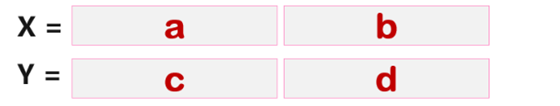分治1复杂度分析：X乘Y，一共有4次位大整数相乘，3次加法，2次移位。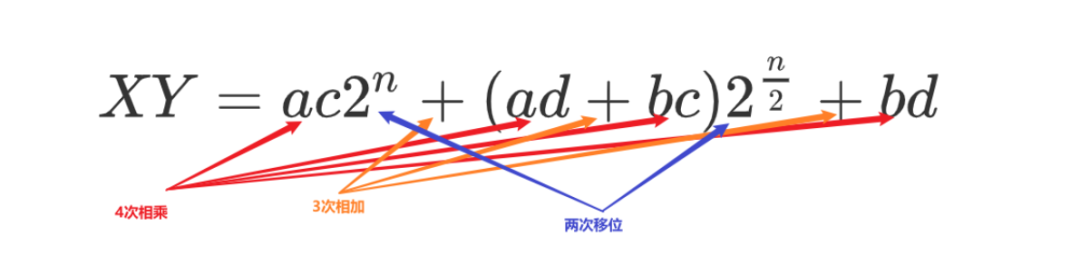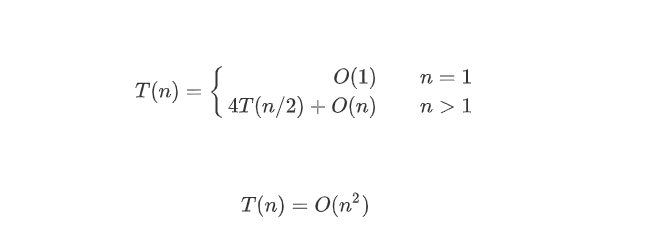这种分治的复杂度与传统计算方法相比并没有改进。分治2复杂度分析：X乘Y，一共有3次位大整数相乘，6次加法，2次移位。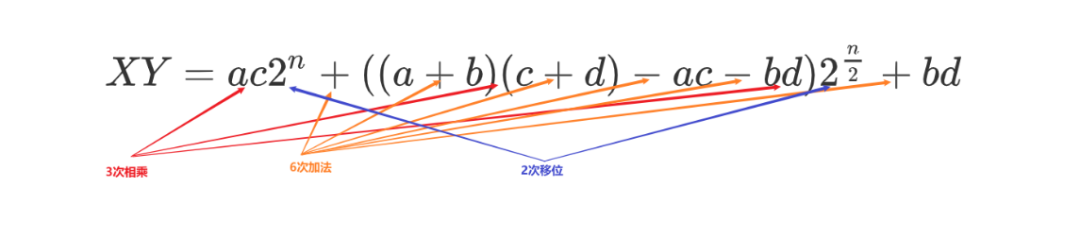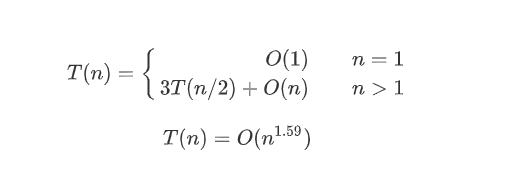相较于传统算法，这种分治算法的时间复杂度有较大的改进。3 C++代码实现#include #include #include #define SIGN(A) ((A > 0) ? 1 : -1)int IntegerMultiply(int X, int Y, int N){ int sign = SIGN(X) * SIGN(Y); int x = abs(X); int y = abs(Y); if((0 == x) || (0 == y))  return 0; if (1 == N)  return x*y; else{  int XL = x / (int)pow(10., (int)N/2);   int XR = x - XL * (int)pow(10., N/2);  int YL = y / (int)pow(10., (int)N/2);  int YR = y - YL * (int)pow(10., N/2);    int XLYL = IntegerMultiply(XL, YL, N/2);  int XRYR = IntegerMultiply(XR, YR, N/2);  int XLYRXRYL = IntegerMultiply(XL - XR, YR - YL, N/2) + XLYL + XRYR;  return sign * (XLYL * (int)pow(10., N) + XLYRXRYL * (int)pow(10., N/2) + XRYR); }}void test2(void){ int x = 1234; int y = 4321; cout<<"x * y = "<4)<<endl;cout<<"x * y = "<endl;    }

展开全文• 看到一道经典的面试题目---大整数相乘问题，完完整整敲了一遍，并且在牛客网运行了一遍，AC过了。 所采用的的方法是进位的方法。 举个例子： 用 789 × 956 ，通过计算我们很容易得到 754284 ， 除了笔算或者口算...
看到一道经典的面试题目---大整数相乘问题，完完整整敲了一遍，并且在牛客网运行了一遍，AC过了。

所采用的的方法是进位的方法。

举个例子：

用 789 × 956 ，通过计算我们很容易得到 754284 ， 除了笔算或者口算的方法我们还可以用一种非常巧妙的方法来进行计算。

这个方法的过程是这样子的：

首先将 9 乘以 789 的每一位数字，得到：                             （ 81 ） （ 72 ）（ 63 ）
然后将 5 乘以 789 的每一位数字，得到：               （ 45 ） （ 40 ） （ 35 ）
最后将 6 乘以 789 的每一位数字，得到：（ 54 ）  （ 48 ） （ 42 ）
各项相加 得到  54 93 163 107 63

得到的数我们将其存放在一个数组里面，这个数组的名字是自定义的，我这里将其定义成ret

ret = 54;

ret = 93;

等等。

开始进行进位操作：

ret --->  93 + 54/10 = 98;

ret = 54%10 = 4；

163 = 163 + 98/10 = 172；

ret = 98%10 = 8;

以此类推：

最后得到的结果为：

ret = 7;

ret = 5;

ret = 4;

ret = 2;

ret = 8;

ret = 4;

这就是我们得到的结果，这只是提供个思路，具体的代码如下：

#include <iostream>
#include <memory.h>
#define SIZE 11000
using namespace std;

//进行两个数相乘，完成以后返回字符串
string multi(int *num1,int size1,int *num2,int size2)
{
string s;
int ret[SIZE];
int i,j,k;
int size = size1+size2;
fill(ret,ret+SIZE,0);

//乘法
//将第二个数组中的一位上的数字分别与第一个数组的所有位相乘
//ret用于进位
for(i = 0;i<size2;i++)
{
k = i;
for(j = 0;j<size1;j++)
ret[k++] += num1[j] * num2[i];
}

//进位求余
for(i = 0;i<=size;i++)
{
ret[i+1] += ret[i]/10;
ret[i] %= 10;
}

if(ret[size])
s+=ret[size]+'0';

for(i=size-1;i>=0;i--)
s+=ret[i]+'0';
return s;
}

int main()
{
string number1,number2;

while(cin>>number1>>number2)
{
int i,j = 0;
int size1,size2;
int num1[SIZE],num2[SIZE];
//将定义的数组初始化为 0
fill(num1,num1+SIZE,0);
fill(num2,num2+SIZE,0);

size1 = number1.size();
size2 = number2.size();

for(i = size1-1;i>=0;i--)
num1[i] = number1[j++] - '0';

i = 0;
for(j = size2-1;j>=0;j--)
num2[j] = number2[i++] - '0';

string s = multi(num1,size1,num2,size2);
if(s=='0')
{
for(i=1;i<=s.size();i++)
cout<<s[i];
cout<<endl;
}
if(s!='0')
cout<<s<<endl;
}
} 


展开全文• 最近在跟coursera上斯坦福大学的算法专项课，其中开篇提到了两个整数相乘问题，其中最简单的方法就是模拟我们小学的整数乘法，可想而知这不是比较好的算法，这门课可以说非常棒，带领我们不断探索更优的算法，然后...
最近在跟coursera上斯坦福大学的算法专项课，其中开篇提到了两个整数相乘的问题，其中最简单的方法就是模拟我们小学的整数乘法，可想而知这不是比较好的算法，这门课可以说非常棒，带领我们不断探索更优的算法，然后介绍可以通过使用分而治之的思想来解决这个问题。下面对该问题的方法以及实现进行介绍。问题定义输入：2个n位的整数x和y输出：x * y如求: 1234567891011121314151617181*2019181716151413121110987654 的结果求解该问题要注意的是由于整数的位数可能超过基本类型的表示范围，所以一种方式是将其转化为字符串进行表示，另一种方式是可以使用一些语言自带的大整数类型(如Java的BigInteger)。参考一些资料才发现，该问题的解法其实有很多种，主要列举以下：１．模拟小学乘法： 竖式乘法累加。２．分治乘法： 最简单的是Karatsuba乘法，一般化以后有Toom-Cook乘法.３．快速傅里叶变换４．中国剩余定理我们主要介绍模拟乘法累加以及使用分治思想的Karatsuba乘法，最后使用Java进行实现。模拟小学乘法7 8 9 6 5 2×         3 2 1 1-----------------7 8 9 6 5 2   7 8 9 6 5 2     ..........       -----------------? ? ? ? ? ? ? ?   如上所示，需要将乘数与被乘数逐位相乘，最后再进行累加，时间复杂度为{O(n^2)}.模拟乘法累加还有一个改进版，上述方法在实现时，每次计算乘法都需要考虑进位，最后在加法时也需要进位，比较麻烦。一种改进的版本如下：9  8×       2  1-------------(9)(8)  (18)(16)     -------------(18)(25)(8)  改进的方法先不算任何的进位，也就是说，将每一位相乘，相加的结果保存到同一个位置，到最后才计算进位。我们可以先将结果保存到一个数组中(不考虑进位)，最后对数组从右向左进行遍历，大于10进行进位。Java实现如下：public class BigNumMul {//simple method 模拟乘法累加public static String bigNumberMul(String num1, String num2) {// 分配一个空间，用来存储运算的结果，num1长的数 * num2长的数，结果不会超过num1+num2长int[] res = new int[num1.length() + num2.length()];// 先不考虑进位问题，根据竖式的乘法运算，num1的第i位与num2的第j位相乘，结果应该存放在结果的第i+j位上for (int i=0; iint a = num1.charAt(i) - ‘0‘;for (int j=0; jint b = num2.charAt(j) - ‘0‘;res[i+j] += a * b;      //max: num1.length()+num2.length()-2}}StringBuilder sb = new StringBuilder();//单独处理进位int carry = 0;//最多就到res.length-2, 最后一个元素没有被占用，还是初始值0for (int k=res.length-2; k >= 0; k--) {int digit = (res[k] + carry) % 10;carry = (res[k] + carry) / 10;sb.insert(0, digit);}if (carry > 0) {sb.insert(0, carry);}String str = sb.toString().replaceFirst("^0*", "");return str.substring(0,str.length()-1);}}显然使用O(n^2)的算法是不够好的，我们应该想一下有没有更好的算法，就像这门课上经常说的一句：Can we do better ?分治：Karatsuba算法分治算法的主要思想是能将问题分解为输入规模更小的子问题，然后递归求解子问题，最后将子问题的结果合并得到原问题的结果，最典型的如归并排序算法。为了得到规模更小的子问题，就要将较大的整数拆分为位数较少的两个整数，参考coursera上的算法专项课，主要计算过程如下：如上图所示，将每个数分别拆分为两部分，分别计算ac, bd, 以及(a+b)(c+d)，最后再减去前面两个，将其组合成最终的结果。我们采用更一般的方式将其表达出来，相应计算方法如下：上述给出了更通用的写法，将ｘ和ｙ同分解后的更小的整数进行表示，最后通过递归的计算ac, ad, bc, bd就可以得到x*y的结果。上述是没有优化过的分治算法，每次递归需要４次乘法，合并结果需要O(n)时间复杂度，所以可以得到时间复杂度的表示：{T(n) = 4T(n/2) + O(n)}通过主方法，可以求得上述时间复杂度为O(n^2)，并没有得到好的改善。Karatsuba算法将上述的４次乘法优化为３次从而减少了时间复杂度。具体过程如下：可以看到上述利用(a+b)(c+d)的结果减去ac和bd得到ad+bc的结果，从而只需要计算三次乘法，其时间复杂度可以表示为：T(n)=3T(n/2)+6n=O(n^{log_{2}3})根据上述算法，使用Java进行实现代码如下：//Karatsuba乘法//此种情况使用long时,数过大可能出现越界,应考虑使用BigIntegerpublic static long karatsuba(long num1, long num2) {//递归终止条件if (num1 < 10 || num2 < 10) {return num1 * num2;}// 计算拆分长度int size1 = String.valueOf(num1).length();int size2 = String.valueOf(num2).length();int halfN = Math.max(size1, size2) / 2;/* 拆分为a, b, c, d */long a = Long.valueOf(String.valueOf(num1).substring(0, size1-halfN));long b = Long.valueOf(String.valueOf(num1).substring(size1-halfN));long c = Long.valueOf(String.valueOf(num2).substring(0, size2-halfN));long d = Long.valueOf(String.valueOf(num2).substring(size2-halfN));// 计算z2, z0, z1, 此处的乘法使用递归long z1 = karatsuba(a, c);long z2 = karatsuba(b, d);long z3 = karatsuba((a + b), (c + d)) - z1 - z2;return (long)(z1 * Math.pow(10, 2*halfN) + z2 + z3 * Math.pow(10, halfN));}注意上述递归的终止条件以及如何表示a, b, c, d. 上述实现使用的是Java中的long类型，但是当整数变大时，使用long类型可能会发生溢出，这里可以使用String来模拟整数的加法及乘法，或者使用Java的BigInteger类型，其实BigInteger内部也是使用的String进行存储，我使用的是BigInteger类型，实现代码如下：//使用BigInteger的karatsuba算法//注意BigInteger的运算没有操作符重载//参考: coursera算法专项1public static BigInteger karatsuba(BigInteger num1, BigInteger num2) {if (num1.compareTo(BigInteger.valueOf(10)) < 0 || num2.compareTo(BigInteger.valueOf(10)) < 0) {return num1.multiply(num2);}int n = Math.max(num1.toString().length(), num2.toString().length());int halfN = n / 2 + n % 2;  //另一种划分方法//返回num1 / halfN 和 num1 % halfNBigInteger[] a_b = num1.divideAndRemainder(BigInteger.valueOf(10).pow(halfN));BigInteger a = a_b;BigInteger b = a_b;BigInteger[] c_d = num2.divideAndRemainder(BigInteger.valueOf(10).pow(halfN));BigInteger c = c_d;BigInteger d = c_d;BigInteger step1 = karatsuba(a, c);BigInteger step2 = karatsuba(b, d);BigInteger step3 = karatsuba(a.add(b), c.add(d));BigInteger step4 = step3.subtract(step2).subtract(step1);   //step3-step2-step1BigInteger res = step1.multiply(BigInteger.valueOf(10).pow(2*halfN)).add(step2).add(step4.multiply(BigInteger.valueOf(10).pow(halfN)));return res;}最后的测试代码如下：public static void main(String[] args) {Scanner sc = new Scanner(System.in);String a = sc.next();String b = sc.next();// 开始计算//String str = BigNumMul.bigNumberMul(a, b);//long res = BigNumMul.karatsuba(Long.valueOf(a), Long.valueOf(b));//String str = Long.toString(res);BigInteger res = BigNumMul.karatsuba(new BigInteger(a), new BigInteger(b));String str = res.toString();System.out.println(a + " * " + b + " = " + str);}总结与感想(1)对一个问题要深入调研和分析，多尝试不同的解决方法。(2)可以多分析一些诸如此类的经典问题，还是比较有意思的。参考资料：1.coursera算法专项2.大数乘法问题及其高效算法3.https://stackoverflow.com/questions/17531042/karatsuba-algorithm-without-biginteger-usage4.https://chenyvehtung.github.io/2017/03/02/about-multiplication.html
展开全文• 分治法求解大整数相乘问题 【问题描述】 设X和Y都是n位的大整数，现在要计算它们的乘积XY。 【提示】采用分治法求解两个十进制大整数的乘法，以提高乘法的效率，减少乘法次数。 计算公式为： XY=AC10n+[(A-B)(D-C)+...
分治法求解大整数相乘问题
【问题描述】 设X和Y都是n位的大整数，现在要计算它们的乘积XY。
【提示】采用分治法求解两个十进制大整数的乘法，以提高乘法的效率，减少乘法次数。
计算公式为：
XY=AC10n+[(A-B)(D-C)+AC+BD]10n/2+BD
下面的例子演示了分治算法的计算过程。
设X=314l，Y=5327，用上述算法计算XY的计算过程可列表如下，其中带’号的数值是在计算完成AC，BD，和(A-B)(D-C)之后才填入的。

X=3141        A=31       B=41        A-B=-10
Y=5327        C=53       D=27        D-C=-26
AC=(1643)’
BD=(1107)’
(A-B)(D-C)=(260)’
XY=(1643)‘104+[(1643)’+(260)’+(1107)’]102+(1107)’
=(16732107)’

A=31        A1=3       B1=1        A1-B1=2
C=53        C1=5       D1=3        D1-C1=-2
A1C1=15     B1D1=3     (A1-B1)(D1-C1)=-4
AC=1500+(15+3-4)10+3=1643

B=41        A2=4       B2=1        A2-B2=3
D=27        C2=2       D2=7        D2-C2=5
A2C2=8     B2D2=7     (A2-B2)(D2-C2)=15
BD=800+(8+7+15)10+7=1107

|A-B|=10        A3=1       B3=0        A3-B3=1
|D-C|=26        C3=2       D3=6        D3-C3=4
A3C3=2     B3D3=0     (A3-B3)(D3-C3)=4
(A-B)(D-C)=200+(2+0+4)10+0=260
【算法时间复杂度分析】
该算法仅需做3次n/2位整数的乘法(AC，BD和(A-B)(D-C))，6次加、减法和2次移位的运算。所以，该算法时间复杂度为：
T(n)=O(nlog3)=O(n1.59)
//代码如下
/*
函数功能：分治法求两个N为的整数的乘积
输入参数：X,Y分别为两个N位整数
算法思想：
时间复杂度为：T(n)=O(nlog3)=O(n1.59)
*/

#include<iostream>
#include<math.h>
using namespace std;

#define SIGN(A) ((A > 0) ? 1 : -1)
int IntegerMultiply(int X, int Y, int N)	//参数为大整数 X，Y及它们相同的位数 N
{
int sign = SIGN(X) * SIGN(Y);			//确定两数乘积的正负
int x = abs(X);							//将两数化为绝对值，之后计算再加入正负号
int y = abs(Y);
if((x == 0) || (y == 0))				//乘数有一个为 0，两数相乘为 0
return 0;
if (N == 1)								//只剩一位时，返回
return x*y;
else
{
int A = x / pow(10, N/2);
int B = x - A * pow(10, N/2);
int C = y / pow(10, N/2);
int D = y - C * pow(10, N/2);

int AC = IntegerMultiply(A, C, N/2);
int BD = IntegerMultiply(B, D, N/2);
int ADBC = IntegerMultiply(A - B, D - C, N/2) + AC + BD;
return sign * (AC * pow(10, N) + ADBC * pow(10, N/2) + BD); //可根据公式化简
}
}

int main()
{
int x, y, z, n = 0;
cin >> x >> y;

z = x;
while(z != 0)			// 求 X，Y有多少位
{
z = z / 10;
++n;
}

cout << "x * y = " << IntegerMultiply(x, y, n) << endl;
cout << "x * y = " << x * y << endl;
return 0;
}



展开全文算法 分治算法
• 求解最大整数相乘问题 题目描述 ： 有两个用字符串表示的非常大的大整数,算出他们的乘积，也是用字符串表示。不能用系统自带的大整数类型。 输入描述: 空格分隔的两个字符串，代表输入的两个大整数 输出...
• 1 问题有两个n位大整数，，它们数值之分大，如。现在要计算它们的乘积... 分治1复杂度分析：X乘Y，一共有4次位大整数相乘，3次加法，2次移位。这种分治的复杂度与传统计算方法相比并没有改进。分治2复杂度分析：X乘...
• 最近在跟coursera上斯坦福大学的算法专项课，其中开篇提到了两个整数相乘问题，其中最简单的方法就是模拟我们小学的整数乘法，可想而知这不是比较好的算法，这门课可以说非常棒，带领我们不断探索更优的算法，然后...
• 最近在跟coursera上斯坦福大学的算法专项课，其中开篇提到了两个整数相乘问题，其中最简单的方法就是模拟我们小学的整数乘法，可想而知这不是比较好的算法，这门课可以说非常棒，带领我们不断探索更优的算法，然后...
• 在Python中本来不存在所谓大整数溢出问题。但是，我看到了一个叫做阿拉伯乘法的方法，是一个古老的计算两个数相乘的问题。于是用它来计算两个大整数相乘，感觉还不错。algorithm python 算法
•c++
• 1 问题有两个n位大整数，，它们数值之分大，如。现在要计算它们的乘积...分治1复杂度分析：X乘Y，一共有4次位大整数相乘，3次加法，2次移位。这种分治的复杂度与传统计算方法相比并没有改进。分治2复杂度分析：X乘Y...java大数乘法分治
• 2.问题分析(1)100位以上的整数，用整数变量直接存储装不下所以，中间运算时，牵扯到大数肯定当做字符串来存储(2)A和B直接乘操作肯定是操作不了，必须是分开来处理可以二分法，将AB转换A=A1*10^(n1/2) +A0 —– n1为a...分治算法 大数相加
• 如题，解决大整数相乘： #include #include #include #include using namespace std; /* 详细描述： char* LongIntegerMul(char* pNum1, char *pNum2); 输入参数： char* pNum1：使用字符串表示的长整数1 ...
• 首先，我们来复习一下分治算法的思想：将一个大的问题，分解成 若干个性质相同或相似的小的问题（最好是独立的），每一个小的问题是可以求解的。再将小的问题，合并成原的大的问题。 而为了解决一个给定的问题，...
• ## 分治法的经典问题——大整数相乘

万次阅读 多人点赞 2016-12-03 17:13:27
通过大整数相乘问题来了解分治法假如现在我们要求两个大整数相乘的乘积，如1234 * 1234（这里为了了分析简便，所以不举形如1234567891234567这样的大整数，不必要在此纠结），那么按照我们小学学的乘法，就是用乘数...分治法 算法 时间复杂度 c++
• 对于大整数计算，一般都要用某种方法转化，否则会溢出。但是python无此担忧了...问题整数相乘思路说明对于大整数计算，一般都要用某种方法转化，否则会溢出。但是python无此担忧了。Python支持“无限精度”的整数...
• 问题整数相乘思路说明对于大整数计算，一般都要用某种方法转化，否则会溢出。但是python无此担忧了。Python支持“无限精度”的整数，一般情况下不用考虑整数溢出的问题，而且Python Int类型与任意精度的Long整数类......